# Deduce the state space representation and state variable matrices A, B, C, and D of the RLC circuit connected with two voltage sources vi(t) & vz(t), as shown in Figure Q3(b). Assume that the outputs are the currents flowing in R1, and R2.

Question-AnswerCategory: Electrical EngineeringDeduce the state space representation and state variable matrices A, B, C, and D of the RLC circuit connected with two voltage sources vi(t) & vz(t), as shown in Figure Q3(b). Assume that the outputs are the currents flowing in R1, and R2.

### Deduce the state space representation and state variable matrices A, B, C, and D of the RLC circuit connected with two voltage sources vi(t) & vz(t), as shown in Figure Q3(b). Assume that the outputs are the currents flowing in R1, and R2.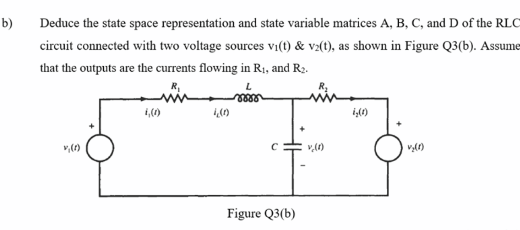Vc and iL are the state variables.

i1 and i2 are the outputs.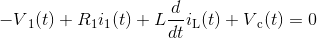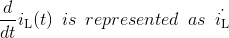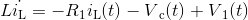(1)
KCL at node: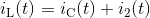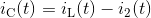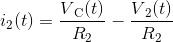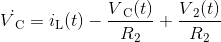(2)
Outputs:
Current through R1 is iL
i1(t) = iL(t) (3)
Current through R2 is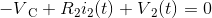(4)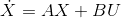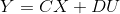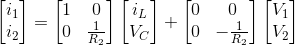————————————————-